GeeksforGeeks App
Open AppBrowser
Continue

# Upper Confidence Bound Algorithm in Reinforcement Learning

In Reinforcement learning, the agent or decision-maker generates its training data by interacting with the world. The agent must learn the consequences of its actions through trial and error, rather than being explicitly told the correct action.

Multi-Armed Bandit Problem

In Reinforcement Learning, we use Multi-Armed Bandit Problem to formalize the notion of decision-making under uncertainty using k-armed bandits. A decision-maker or agent is present in Multi-Armed Bandit Problem to choose between k-different actions and receives a reward based on the action it chooses. Bandit problem is used to describe fundamental concepts in reinforcement learning, such as rewards, timesteps, and values.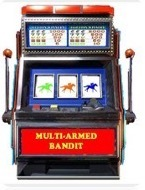The picture above represents a slot machine also known as a bandit with two levers. We assume that each lever has a separate distribution of rewards and there is at least one lever that generates maximum reward.

The probability distribution for the reward corresponding to each lever is different and is unknown to the gambler(decision-maker). Hence, the goal here is to identify which lever to pull to get the maximum reward after a given set of trials.

For Example: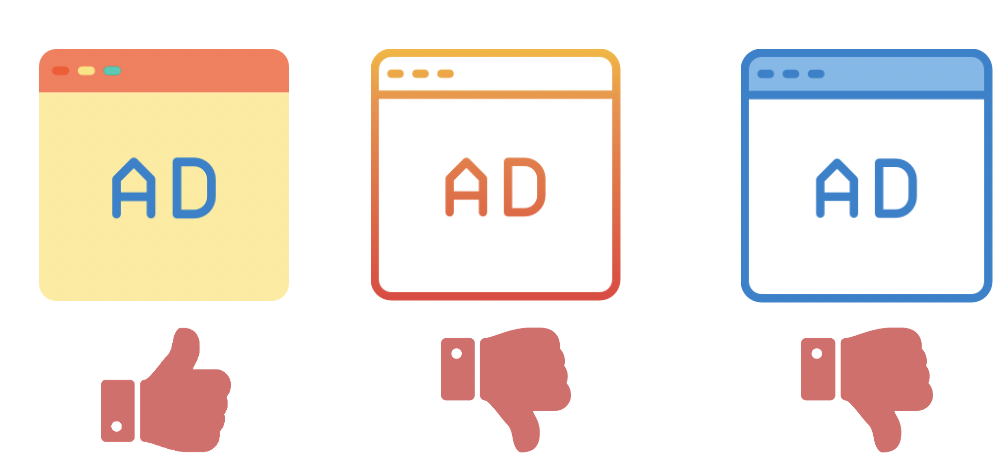If the advertiser only displays one ad, then he can no longer collect data on the other two ads. Perhaps one of the other ads is better, it only appears worse due to chance. If the other two ads are worse, then continuing the study can affect the click-through rate adversely. This advertising trial exemplifies decision-making under uncertainty.
In the above example, the role of the agent is played by an advertiser. The advertiser has to choose between three different actions, to display the first, second, or third ad. Each ad is an action. Choosing that ad yields some unknown reward. Finally, the profit of the advertiser after the ad is the reward that the advertiser receives.

Action-Values:

For the advertiser to decide which action is best, we must define the value of taking each action. We define these values using the action-value function using the language of probability. The value of selecting an action q*(a) is defined as the expected reward Rt we receive when taking an action a from the possible set of actions.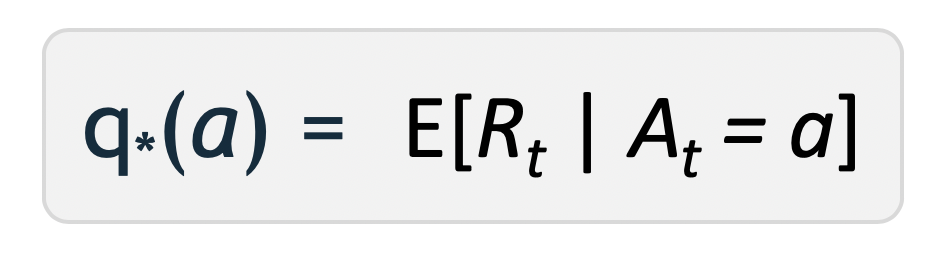The goal of the agent is to maximize the expected reward by selecting the action that has the highest action-value.

Action-value Estimate:

Since the value of selecting an action i.e. Q*(a) is not known to the agent, so we will use the sample-average method to estimate it.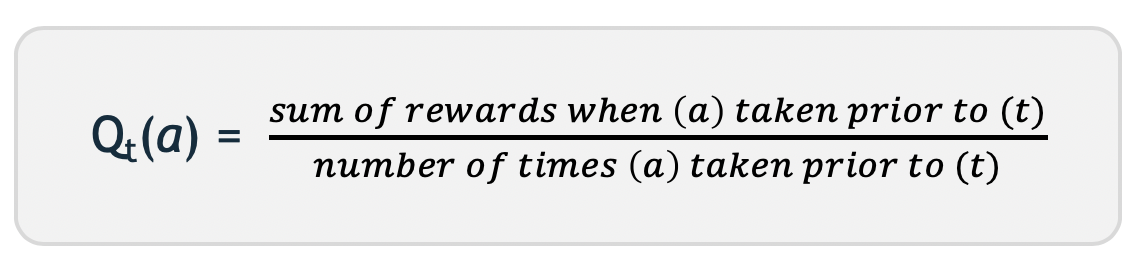Exploration vs Exploitation:

• Greedy Action: When an agent chooses an action that currently has the largest estimated value. The agent exploits its current knowledge by choosing the greedy action.
• Non-Greedy Action: When the agent does not choose the largest estimated value and sacrifice immediate reward hoping to gain more information about the other actions.
• Exploration: It allows the agent to improve its knowledge about each action. Hopefully, leading to a long-term benefit.
• Exploitation: It allows the agent to choose the greedy action to try to get the most reward for short-term benefit. A pure greedy action selection can lead to sub-optimal behaviour.

A dilemma occurs between exploration and exploitation because an agent can not choose to both explore and exploit at the same time. Hence, we use the Upper Confidence Bound algorithm to solve the exploration-exploitation dilemma

Upper Confidence Bound Action Selection:
Upper-Confidence Bound action selection uses uncertainty in the action-value estimates for balancing exploration and exploitation. Since there is inherent uncertainty in the accuracy of the action-value estimates when we use a sampled set of rewards thus UCB uses uncertainty in the estimates to drive exploration.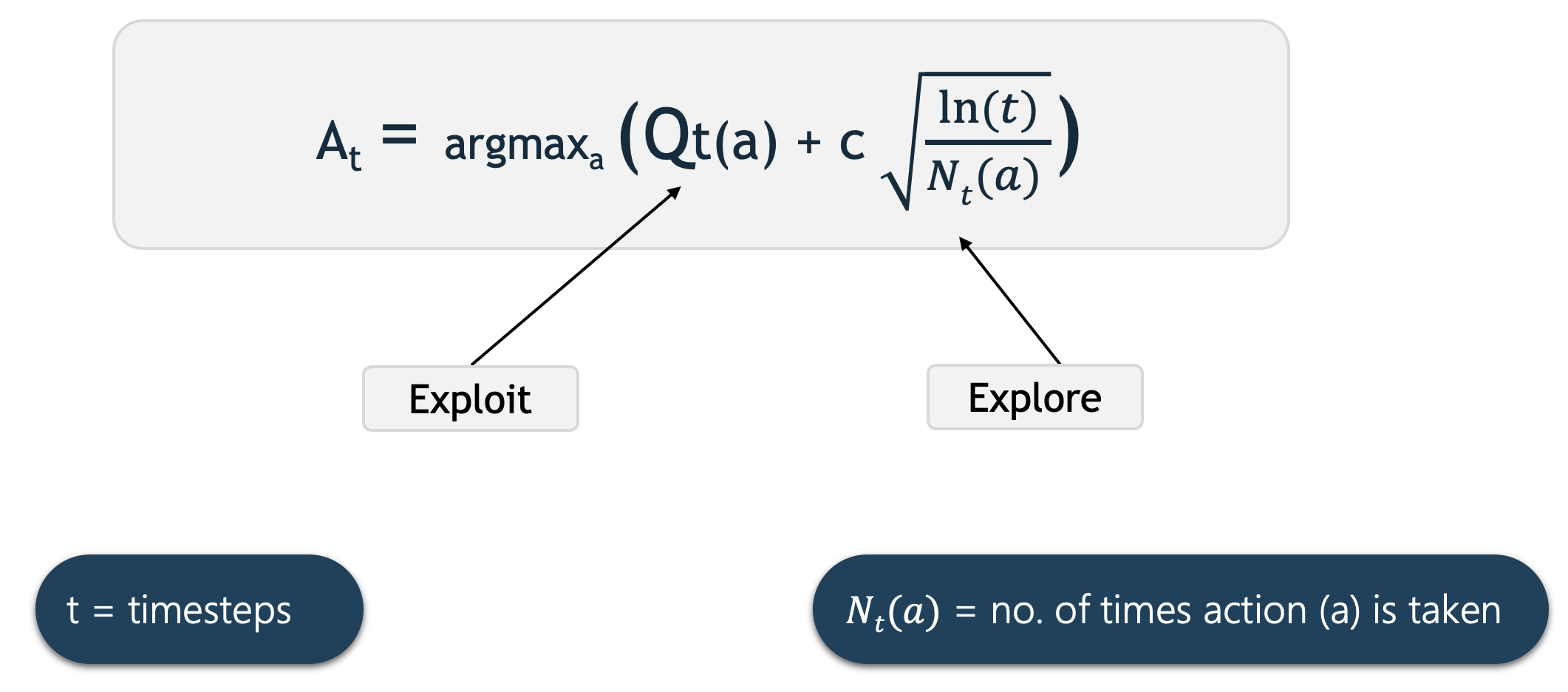Qt(a) here represents the current estimate for action a at time t. We select the action that has the highest estimated action-value plus the upper-confidence bound exploration term.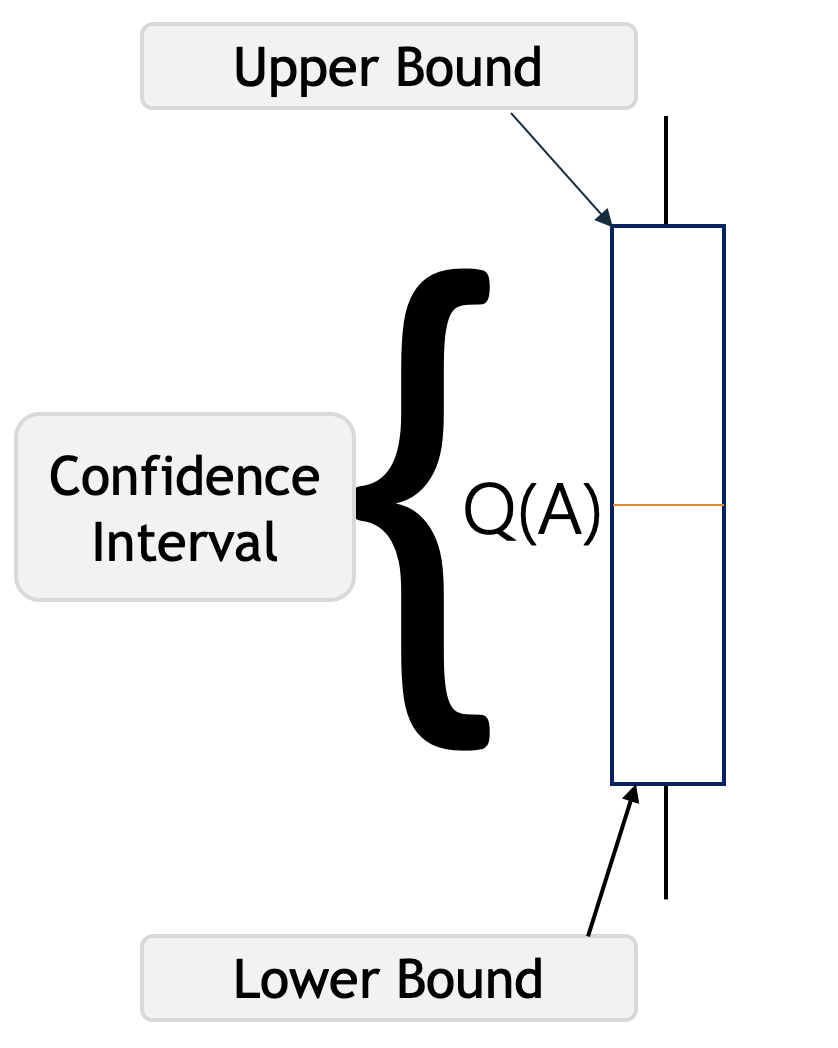Q(A) in the above picture represents the current action-value estimate for action A. The brackets represent a confidence interval around Q*(A) which says that we are confident that the actual action-value of action A lies somewhere in this region.

The lower bracket is called the lower bound, and the upper bracket is the upper bound. The region between the brackets is the confidence interval which represents the uncertainty in the estimates. If the region is very small, then we become very certain that the actual value of action A is near our estimated value. On the other hand, if the region is large, then we become uncertain that the value of action A is near our estimated value.

The Upper Confidence Bound follows the principle of optimism in the face of uncertainty which implies that if we are uncertain about an action, we should optimistically assume that it is the correct action.

For example, let’s say we have these four actions with associated uncertainties in the picture below, our agent has no idea which is the best action. So according to the UCB algorithm, it will optimistically pick the action that has the highest upper bound i.e. A. By doing this either it will have the highest value and get the highest reward, or by taking that we will get to learn about an action we know least about.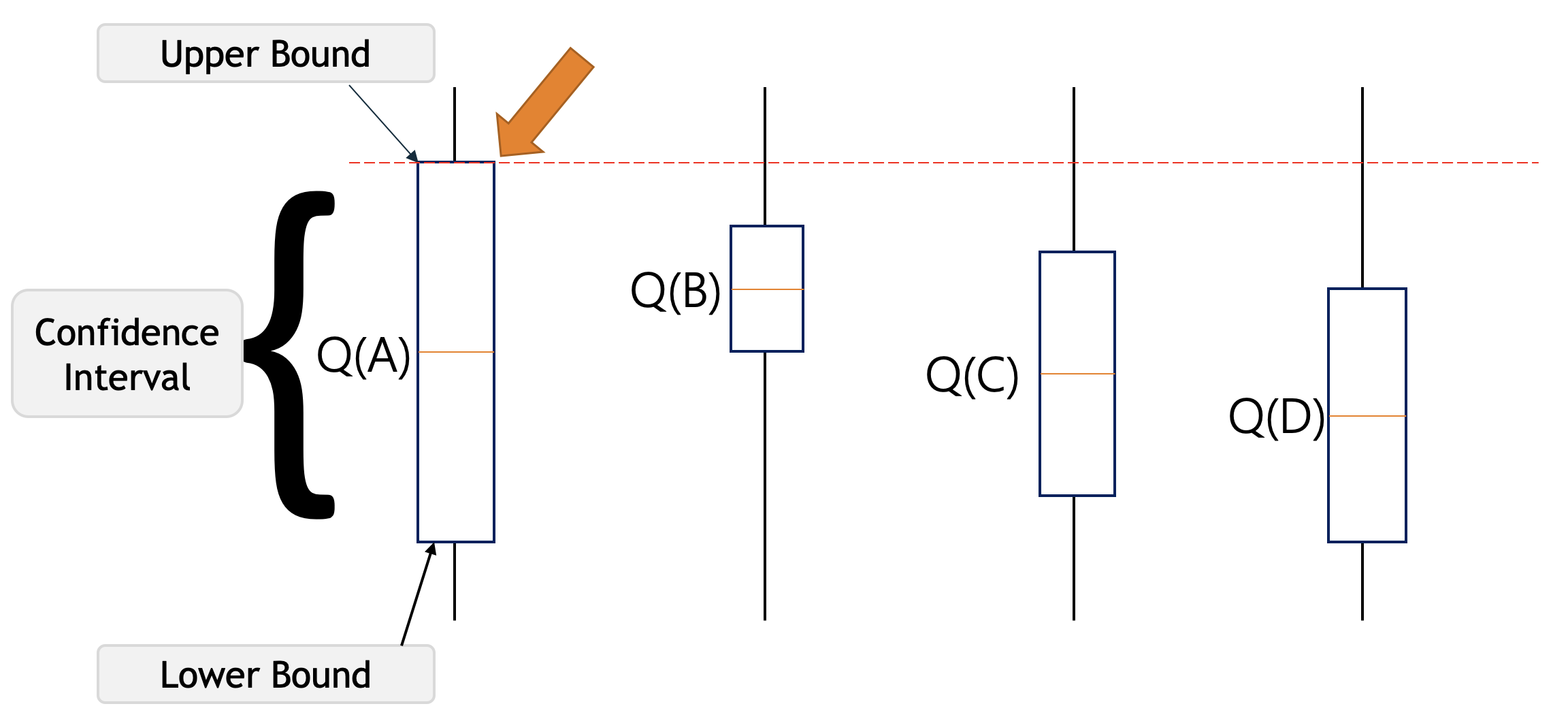Let’s assume that after selecting the action A we end up in a state depicted in the picture below. This time UCB will select the action B since Q(B) has the highest upper-confidence bound because it’s action-value estimate is the highest, even though the confidence interval is small.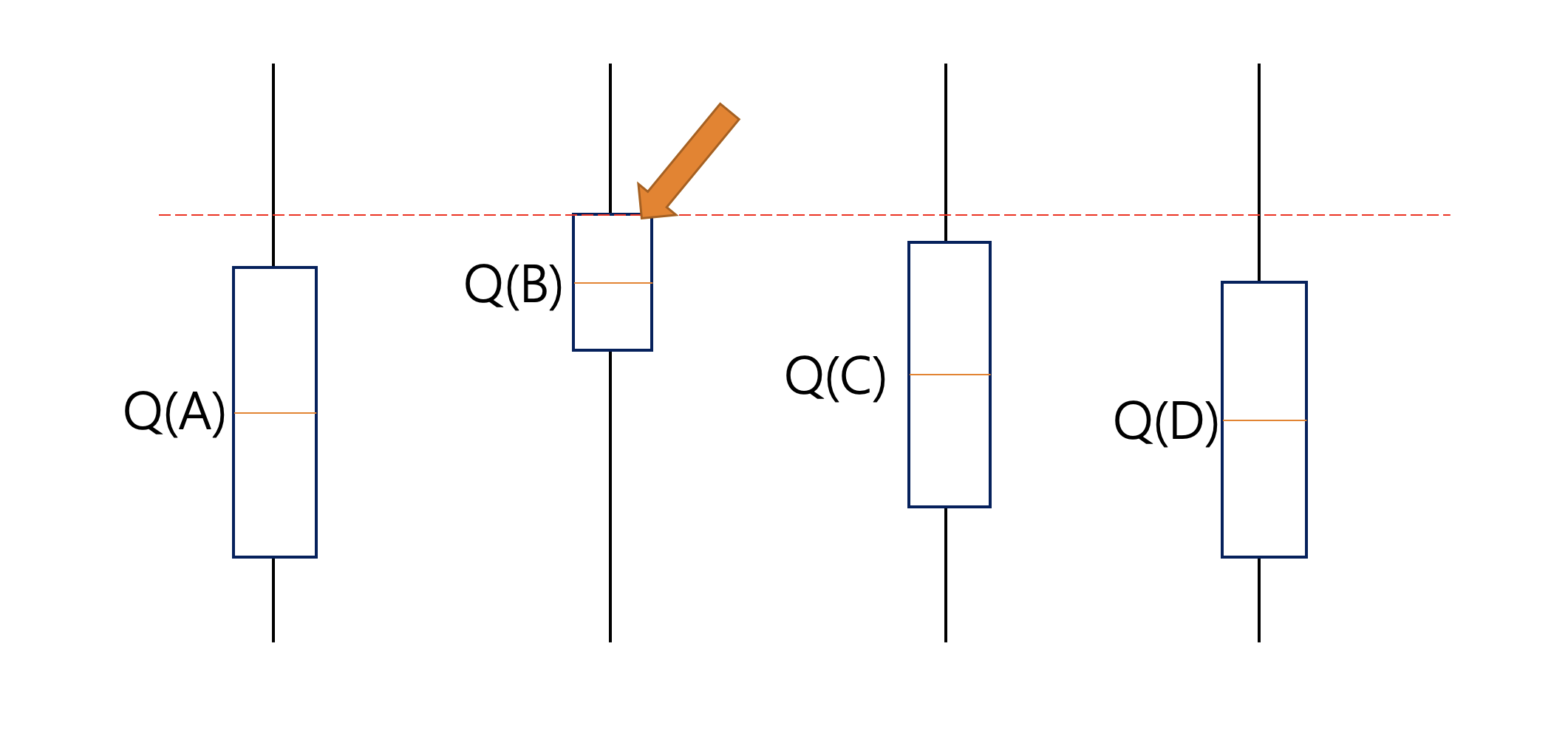Initially, UCB explores more to systematically reduce uncertainty but its exploration reduces over time. Thus we can say that UCB obtains greater reward on average than other algorithms such as Epsilon-greedy, Optimistic Initial Values, etc.

My Personal Notes arrow_drop_up